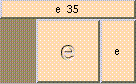ETHE BOX'S MAIN HELP: This is the constant e ~ 2.718281828459 e denotes the unquie positive real number such that log(e) = 1. e (output): This is the constant e ~ 2.718281828459 e denotes the unquie positive real number such that log(e) = 1.

<-- BACK TO Math